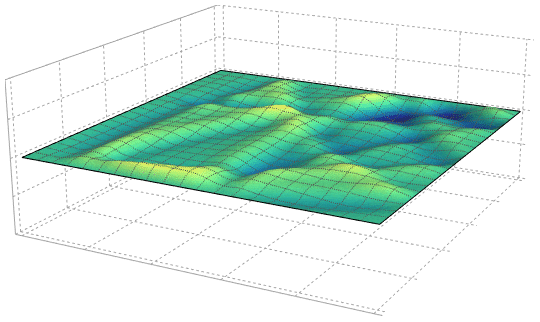## PhotoPhoto

## PhotoPhoto

## Alternating wave pattern on RopesI saw someone working out with…## Alternating wave pattern on Ropes

I saw someone working out with Battle Ropes the other day and this wonderful pattern emerged was absolutely fascinating. Of course, the waveforms are not purely sinusoidal but it helps us to understand why you see such patterns

## fuckyeahphysica: The simple harmonic oscillator Anonymous asked:…## The simple harmonic oscillator

Okay Anon! Here you go, this is my rendition.

## The problem

You have a mass suspended on a spring. We want to know where the mass will be at any instant of time.

Describe the motion of the mass

## The physical solution

Now before we get on to the math, let us first visualize the motion by attaching a spray paint bottle as the mass.

Oh, wait that seems like a function that we are familiar with – The sinusoid.

Without even having to write down a single equation, we have found out the solution to our problem. The motion that is traced  by the mass is a sinusoid.

## But what do I mean by a sinusoid ?

If you took the plotted paper and tried to create that function with the help of sum of polynomials i.e x, x2, x3 … Now you this what it would like :

By taking an infinite of these polynomial sums you get the function Since this series of polynomial occurs a lot, its given the name – sine.

I hope this shed some light on the intuition of the SHM equation. Have fun!

## A note on the cosine

For people asking about the cosine and how to think about it . Cosine is merely sine pushed to the side.

The analogy works the same way 😀

Have a good one!## The simple harmonic oscillator

Okay Anon! Here you go, this is my rendition.

## The problem

You have a mass suspended on a spring. We want to know where the mass will be at any instant of time.

Describe the motion of the mass

## The physical solution

Now before we get on to the math, let us first visualize the motion by attaching a spray paint bottle as the mass.

Oh, wait that seems like a function that we are familiar with – The sinusoid.

Without even having to write down a single equation, we have found out the solution to our problem. The motion that is traced  by the mass is a sinusoid.

## But what do I mean by a sinusoid ?

If you took the plotted paper and tried to create that function with the help of sum of polynomials i.e x, x2, x3 … Now you this what it would like :

By taking an infinite of these polynomial sums you get the function Since this series of polynomial occurs a lot, its given the name – sine.

I hope this shed some light on the intuition of the SHM equation. Have fun!